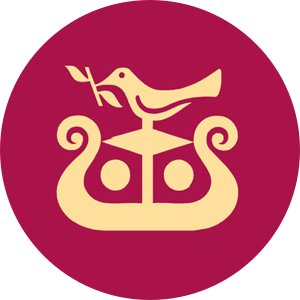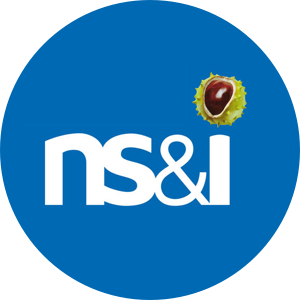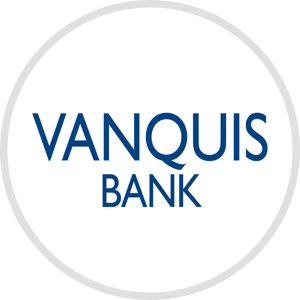# Supported banks / providers

## Did you know Money Dashboard supports over 70 UK banks? Check out the full list below and get connected today or request a provider if yours isn't listed.BetaBetaBetaBetaBetaBetaBetaBetaBetaBetaBetaBetaBetaBetaBetaBetaBetaBetaBetaBetaBetaBetaBetaBetaBetaBetaBetaBetaBetaBetaBetaBetaBetaBetaBetaBetaBetaBetaBetaBetaBetaBetaBetaBetaBetaBetaBetaBetaBetaBetaBetaBetaBetaBetaBetaBetaBetaBetaBetaBetaBetaBetaBetaBetaBetaBetaBetaBetaBetaBetaBetaBetaBetaBetaBetaBetaBetaBetaBetaBetaBetaBetaBetaBetaBetaBetaBetaBetaBetaBetaBetaBetaBetaBetaBetaBeta